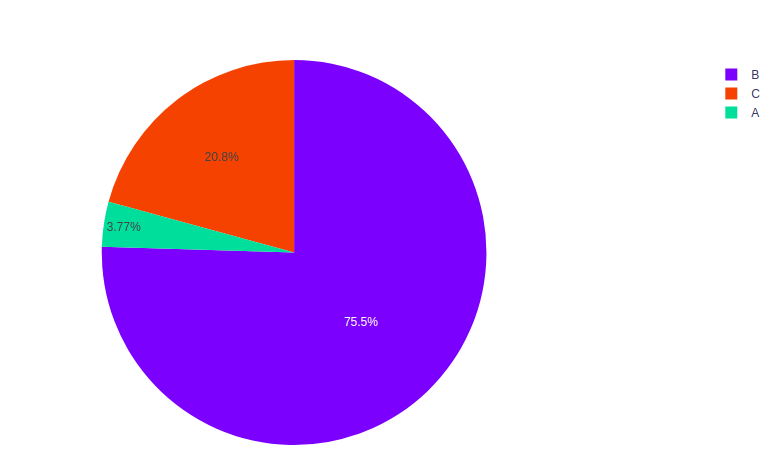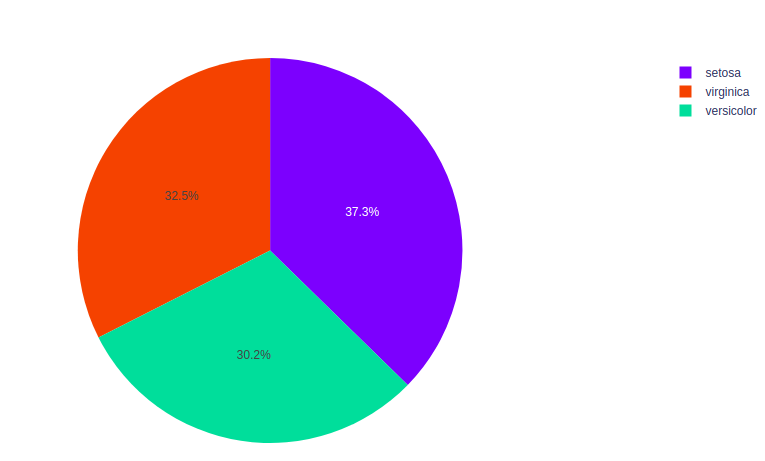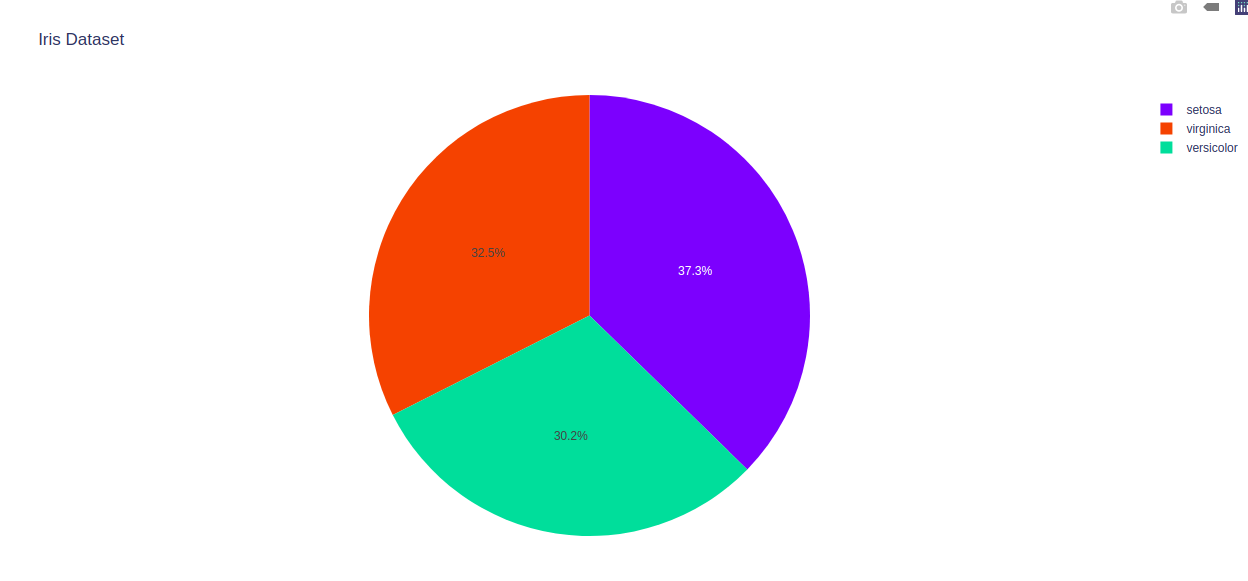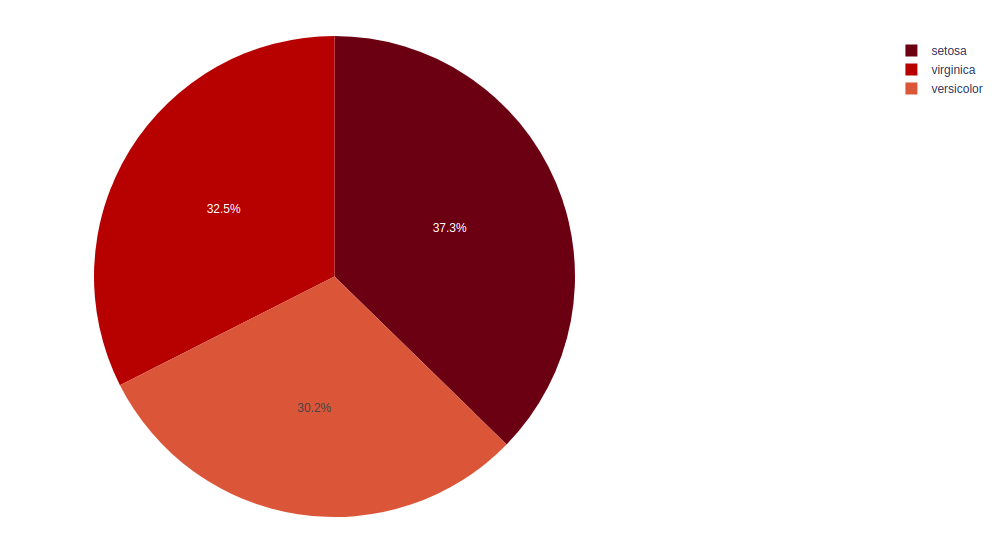GeeksforGeeks App
Open AppBrowser
Continue

# Pie plot using Plotly in Python

Plotly is a Python library which is used to design graphs, especially interactive graphs. It can plot various graphs and charts like histogram, barplot, boxplot, spreadplot and many more. It is mainly used in data analysis as well as financial analysis. plotly is an interactive visualization library.

## Pie Plot

A pie chart is a circular analytical chart, which is divided into region to symbolize numerical percentage. In px.pie, data anticipated by the sectors of the pie to set the values. All  sector  are classify in names. Pie chart is used usually to show the percentage with next corresponding slice of pie. Pie chart helps to make understand well because of its different portions and color codings.

Syntax: plotly.express.pie(data_frame=None, names=None, values=None, color=None, color_discrete_sequence=None, color_discrete_map={}, hover_name=None, hover_data=None, custom_data=None, labels={}, title=None, template=None, width=None, height=None, opacity=None, hole=None)

Parameters:

Example:

## Python3

 `import` `plotly.express as px``import` `numpy` `# Random Data``random_x ``=` `[``100``, ``2000``, ``550``]``names ``=` `[``'A'``, ``'B'``, ``'C'``]` `fig ``=` `px.pie(values``=``random_x, names``=``names)``fig.show()`

Output:## Grouping Data

The same value for the names parameter are grouped together. Repeated labels visually groups rows or columns together to make the data easier to understand. Let’s see one example given below.

Example: The iris dataset contains many rows but only 3 species so the data is grouped according to the species.

## Python3

 `import` `plotly.express as px` `# Loading the iris dataset``df ``=` `px.data.iris()` `fig ``=` `px.pie(df, values``=``"sepal_width"``, names``=``"species"``)``fig.show()`

Output:## Customizing pie chart

The pie chart can be customized by using the px.pie, using some of its parameters such as hover_data and labels. Let’s see the below example for better understanding.

Example:

## Python3

 `import` `plotly.express as px` `# Loading the iris dataset``df ``=` `px.data.iris()` `fig ``=` `px.pie(df, values``=``"sepal_width"``, names``=``"species"``,``             ``title``=``'Iris Dataset'``, hover_data``=``[``'sepal_length'``])``fig.show()`

Output:## Setting Colors

The color of pie can be change in plotly module. Different colors help in to distinguish the data from each other, which help  to understand the data more efficiently.

Example:

## Python3

 `import` `plotly.express as px` `# Loading the iris dataset``df ``=` `px.data.iris()` `fig ``=` `px.pie(df, values``=``"sepal_width"``,``             ``names``=``"species"``,``             ``color_discrete_sequence``=``px.colors.sequential.RdBu)``fig.show()`

Output:My Personal Notes arrow_drop_up